Tamilnadu State Board New Syllabus Samacheer Kalvi 12th Maths Guide Pdf Chapter 10 Ordinary Differential Equations Ex 10.3 Textbook Questions and Answers, Notes.

## Tamilnadu Samacheer Kalvi 12th Maths Solutions Chapter 10 Ordinary Differential Equations Ex 10.3

Question 1.
Find the differential equation of the family of (i) all non-vertical lines in a plane
(ii) all non horizontal lines in a plane.
Solution:
(i) The equation of the family of non vertical lines in a plane ax + by = 1, b ≠ 0, a ∈ R
Given equation is ax + by = 1 …….. (1)
Differentiating equation (1) with respect to ‘x’ we get
a + b $$\frac { dy }{ dx }$$ = 0
∵ 2 arbitrary constant,
∴ Differentiating twice continuously
Again differentiating above equation with respect to ‘x’, we get
0 + b $$\frac { d^2y }{ dx^2 }$$ = 0
$$\frac { d^2y }{ dx^2 }$$ = 0 [∵ b ≠ 0]
$$\frac { d^2y }{ dx^2 }$$ = 0 is a required differential equation.

(ii) The equation of the family of non horizontal
lines in a,plane ax + by = 1, a ≠ 0 & b ∈ R
Given equation is ax + by = 1 ……… (1)
Differentiating equation (1) with respect to ‘y’, we get
a $$\frac { dx }{ dy }$$ + b = 0
∵ 2 arbitrary constant,
∴ Differentiating twice continuously
Again differentiating we get
a $$\frac { d^2x }{ dy^2 }$$ = 0 [∵ a ≠ 0]
$$\frac { d^2x }{ dy^2 }$$ = 0 is a required differential equation.Question 2.
Form the differential equation of all straight lines touching the circle x² + y² = r²
Solution:
Given circle equation be x² + y² = r²
Let y = mx + c be all straight lines which towards the given circle x² + y² = r²
The condition for y = mx + c ……. (1)
be a tangent to the circle x² + y² = r²
be c² = r² (1 + m²) ⇒ c = r $$\sqrt { 1+m^2 }$$
Substituting c value in equation (1), we get
y = mx + r $$\sqrt { 1+m^2 }$$
y – mx = r$$\sqrt { 1+m^2 }$$ ……. (2)
Differentiating equation (2) w.r.t x, we get
$$\frac { dy }{ dx }$$ – m = 0
$$\frac { dy }{ dx }$$ = m …….. (3)
substituting equation (3) in equation (2), we get;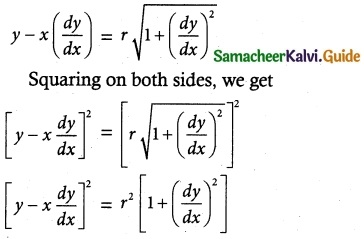Which is a required differential equation.Question 3.
Find the differential equation of the family of circles passing through the origin and having their centres on the x-axis.
Solution:
Given the circles centre on x-axis & the circle is passing through the origin.
Let it be (r, 0) & its radius r.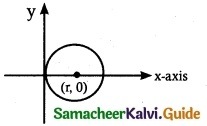Equation of the circle is
(x – a)² + (y – b)² = r²
(x – r)² + (y – 0)² = r²
x² – 2xr + r² + y² = r²
x² – 2xr + y² = r² – r²
x² – 2xr + y² = 0 ……. (1)
Differentiating equation (1) with respect to ‘x’, we get
2x – 2r + 2y $$\frac { dy }{ dx }$$ = 0 dx
2x + 2y$$\frac { dy }{ dx }$$ = 2r
x + y$$\frac { dy }{ dx }$$ = r
Substituting r value in equation (1), we get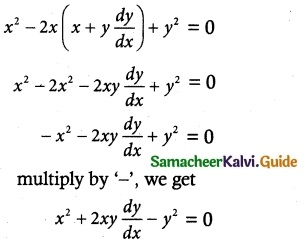Which is a required differential equation.Question 4.
Find the differential equation of the family of all the parabolas with latus rectum 4a and whose axes are parallel to the x-axis.
Solution:
Given the equation of family of parabolas with latus rectum 4a and axes are parallel to x-axis then
(y – b)² = 4a (x – a), where (a, b) is the vertex of parabola.
y² – 2yb + b² = 4ax – 4a² ……. (1)
Differentiating equation (1) with respect to x, we get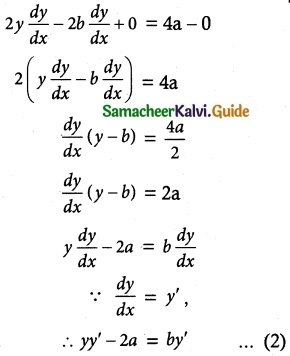Differentiating equation (2) with respect to ‘x’, we get
yy”+ y’y’ = by”
yy” + y’2 = by” ……. (3)
Substituting the b value in (3), we get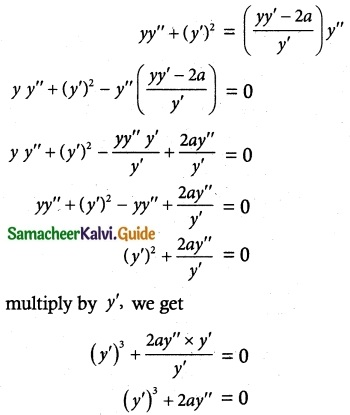Which is a required differential equation.Question 5.
Find the differential equation of the family of parabolas with vertex at (0, -1) and having axis along the y axis.
Solution:
Equation of the family of parabolas with vertex at (0, -1) and having axis along the y-axis is
(x – 0)² = 4a(y + 1)
x² = 4a (y + 1) ……. (1)
x² = 4 ay + 4a
Differentiating equation (1) with respect to ‘x’, we get
2x = 4a y’
$$\frac { 2x }{ y’ }$$ = 4a
Substituting 4a value in equation (1), we get
x² = $$\frac { 2x }{ y’ }$$(y + 1)
$$\frac { x^2 }{ x }$$ = $$\frac { 2 }{ y’ }$$(y + 1)
x = $$\frac { 2 }{ y’ }$$ (y + 1)
xy’ = 2 (y + 1)
xy’ = 2y + 2
xy’ – 2y – 2 = 0 is a required differential equation.Question 6.
Find the differential equations of the family of all the ellipses having foci on the y-axis and centre at the origin.
Solution:
The equation of the family of ellipses having centre at the origin & foci on the y-axis, is given by $$\frac { x^2 }{ a^2 }$$ + $$\frac { y^2 }{ b^2 }$$ = 1 ……… (1)
where b > a & a, b are the parameters or a,b are arbitrary constant.
Differentiating equation (1) twice successively, (because we have two arbitrary constant) we get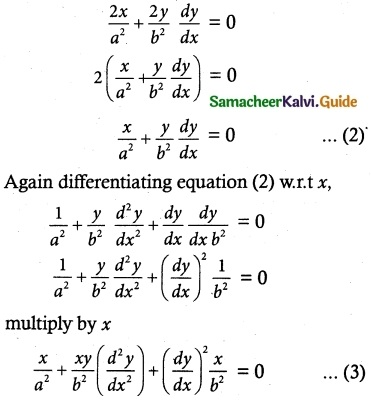Equ (3) – (2) we get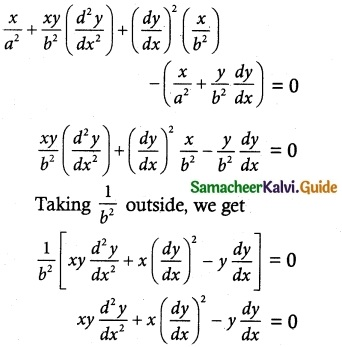is the required differential equation.Question 7.
Find the differential equation corresponding to the family of curves represented by the equation y = Ae8x + Be -8x, where A and B are arbitrary constants.
Solution:
Given y = Ae8x + Be-8x …….. (1)
where A & B are aribitrary constants differentiating equation (1) twice successively (because we have two arbitrary constant), we get
$$\frac { dy }{ dx }$$ = Ae8x + Be-8x (-8)
$$\frac { dy }{ dx }$$ = 8Ae8x – 8Be-8x ……… (2)
$$\frac { d^2y }{ dx^2 }$$ = 8Ae8x (8) – Be-8x (-8)
= 64Ae8x + 64Be-8x
= 64[Ae8x + Be-8x] ……….. (3)
Substituting eqn (1) in eqn (3), we get
$$\frac { d^2y }{ dx^2 }$$ = 64 y
$$\frac { d^2y }{ dx^2 }$$ – 64 y = 0 is the required differential equation.Question 8.
Find the differential equation of the curve represented by xy = aex + be-x + x²
Solution:
Given xy = aex + be-x + x² ……… (1)
where a & b are aribitrary constant,
differentiate equation (1) twice successively,
because we have two arbitray constant.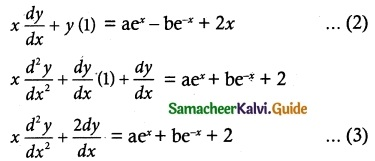From (1), we get xy – x² = aex + be-x …….. (4)
Substituting equation (4) in (3), we get
∴ x $$\frac { d^2y }{ dx^2 }$$ + $$\frac { 2dy }{ dx }$$ – xy + x² – 2 = 0 is the required differential equation.